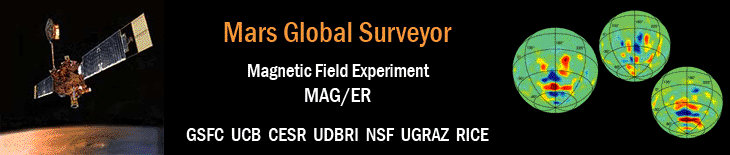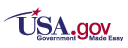'# Tectonic implications of Mars crustal magnetismMap Data

### Connerney, J.E.P., M.H. Acuña, N.F. Ness, G. Kletetschka, D.L. Mitchell, R.P. Lin, and H. Rème

Proceedings of the National Academy of Sciences,

Vol. 102, Iss. 42, pp. 14970-14975, doi: 10.1073/pnas.0507469102, 18 Oct 2005.

For positions near the poles (latitudes between -87 degrees and -90 degress, and between 85 degrees and 90 degrees) the filtered field value (∆Br) may equal zero NT because the spacecraft's orbit does not cover those areas, so sampling was not possible.

## Mars Global Surveyor MAG/ER Maps - Ascii File

The file pnas_102_42_connerney_dbr_map_data.txt contains the data available in the radial map in terms of filtered B-field component, ∆Br, and location of the spacecraft in the order r, latitude, and east longitude.

## Mars Global Surveyor MAG/ER Maps - IDL Files

The files am8e8_dbr_360x180.bin or am8e8_dbr_360x180_pc.bin contain IDL unformatted data array of the maps of the filtered radial (∆Br) component of the vector magnetic field for Mars.

The following IDL commands will allow the user to read the file:

```IDL> dbr = fltarr(360,180)
IDL> openr, lun, 'am8e8_dbr_360x180.bin', /get_lun
IDL> free_lun, lun
```
Each value in the data array represents the median for all data within an 1 degree by 1 degree bin. The array is arranged with the first index equal to the minimum east longitude in degrees - ranging from 0 degrees to 359 degrees - of the bin. The second index is related to the latitude where index 0 is the bin from -90 degrees to and including -89 degrees and index 179 is the bin from +89 degrees to and including +90 degrees (i.e., second index = minimum latitude + 90).

A couple examples are

• for longitude bin 171 degrees through 172 degrees and
latitude bin -55 degrees through -54 degrees
• center longitude is 171.5 degrees and center latitude is -54.5 degrees
• data would be at the indices (171,35) with dbr(171,35) = -25.420 nT
• for longitude bin 200 degrees through 201 degrees and
latitude bin 45 degrees through 46 degrees
• center longitude is 200.5 degrees and center latitude is 45.5 degrees
• data would be at the indices (200,135) with dbr(200,135) = 0.163 nT

## From Appendix A: Magnetic Field Map.

Magnetic fields generated by the solar wind interaction are greater in magnitude near the sub-solar point and much smaller over the darkened hemisphere. They are also global in spatial scale and therefore highly correlated along track. Components of the magnetic field measured on repeat passes over the same location often appear similar, differing only by a constant offset or long wavelength component attributable to the external field. The map was constructed using night side observations of the radial field component, averaged and decimated along track, producing a time series of Br sampled once every degree latitude traversed by MGS. We then applied a differentiating filter (three point Lanczos non-recursive) to the radial field component series, attenuating low frequency signals associated with large spatial scales, and sorted into bins (1° latitude by 1° longitude) from which the median value was extracted as an estimate of the crustal field.

The median value of this quantity (∆Br) is an estimate of the change in the radial magnetic field along track, approximately in the theta direction, as the spacecraft traverses 1° of latitude. ∆Br is relatively insensitive to external fields and closely related to the theta component of the field (see below). The resulting map has about an order of magnitude increased sensitivity to variations in crustal magnetization.

∆Br. The components of the vector field (Br, Bθ, Bφ) may be obtained from the gradient of a scalar potential function, V, represented by a spherical harmonic expansion:

V = a Σ (a/r)n+1 Σ Pnm (cos θ) [gnm cos(mφ) + hnm sin(mφ)],

where a is the planet radius, θ is colatitude, φ is longitude, and the Pnm are the associated Legendre functions of degree n and order m with Schmidt normalization. The gnm and hnm are spherical harmonic coefficients of degree n and order m. The radial component of the field is the directional derivative of the potential in the radial direction, Br = - ∂V/∂r and the theta component is the directional derivative of the potential in the theta direction, Bθ = - 1/r ∂V/∂ θ. The quantity ∆Br computed along track closely approximates ∂ Br/∂ θ, which differs from Bθ only by the appearance of an additional factor - (n + 1) in the expansion of Bθ by harmonics of degree n. The crustal fields measured at orbit altitude (h) are characterized by large n, and a fairly narrow range of n. The satellite altitude acts as a filter that removes wavelengths much larger than h (corresponding to small values of n) and wavelengths much smaller than h (corresponding to large values of n). Measured signal amplitudes are greatest at wavelengths compar able to the altitude of observation (e.g., 38, 8) so we may consider the significant terms in the expansion of ∆Br to be nearly the same as those of an appropriately scaled Bθ. Thus ∆Br appears much like Bθ, apart from the reversal in sign. This may be verified by comparison of contours in Figure 1 with those of Bθ from previous maps (6). ∆Br may be regarded as a proxy for Bθ, acquired in a manner that beneficially supresses unwanted noise due to externally generated fields. Satellite studies of the Earths magnetic field often use high-pass filtered component data (39) but we prefer ∆Br as computed here by virtue of its close association with Bθ .